Custom SearchCHAPTER 19 NUMERICAL TRIGONOMETRY The word "trigonometry" means "measurement by triangles." As it is presented in many textbooks, trigonometry includes topics other than triangles and measurement. However, this chapter is intended only as an introduction to the numerical aspects of trigonometry as they relate to measurement of lengths and angles. SPECIAL PROPERTIES OF RIGHT TRIANGLES A RIGHT TRIANGLE has been defined as any triangle containing a right angle. The side opposite the right angle in a right triangle is a HYPOTENUSE. (See fig. 19- 1.) In figure .19-l, side AC is the hypotenuse.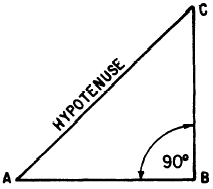Figure 19-1.-A right triangle. An important property of all right triangles, which relates the lengths of the three sides, was discovered by the Greek philosopher Pythagoras. PYTHAGOREAN THEOREM The rule of Pythagoras, or PYTHAGOREAN THEOREM, states that the square of the length of the hypotenuse (in any right triangle) is equal to the sum of the squares of the lengths of the other two sides. For example, if the sides are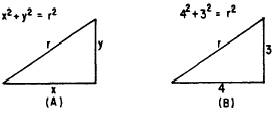Figure 19-2.-The Pythagorean Theorem. (A) General triangle; (B) triangle with sides of specific lengths. labeled as in figure 19-2 (A), the Pythagorean Theorem is stated in symbols as follows: x2 + y2 = r2 An example of the. use of the Pythagorean Theorem in a problem follows: EXAMPLE: Find the length of the hypotenuse in the triangle shown in figure 19-2 (B). SOLUTION: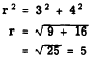EXAMPLE: An observer on a ship at point A, figure 19-3, knows that his distance from point C is 1,200 yards and that the length of BC is 1,300 yards. He measures angle A and finds that it is 90. Calculate the distance from A to B. SOLUTION: By the rule of Pythagoras,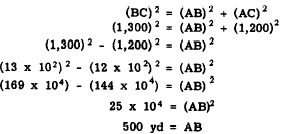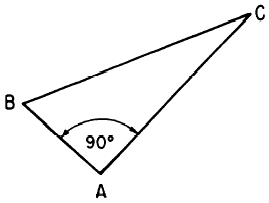Figure 19-3.-Using the Pythagorean Theorem.Integrated Publishing, Inc. - A (SDVOSB) Service Disabled Veteran Owned Small Business